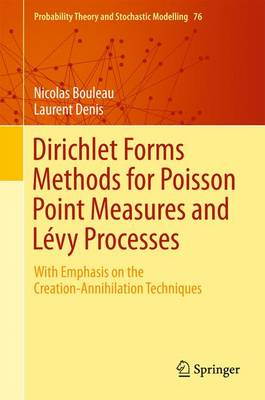•# Dirichlet Forms Methods for Poisson Point Measures and Levy Processes: With Emphasis on the Creation-Annihilation Techniques - Probability Theory and Stochastic Modelling 76 (Hardback)

(author), (author)
£99.99
Hardback 323 Pages / Published: 23/12/2015
• We can order this

Usually dispatched within 3 weeks

A simplified approach to Malliavin calculus adapted to Poisson random measures is developed and applied in this book. Called the "lent particle method" it is based on perturbation of the position of particles. Poisson random measures describe phenomena involving random jumps (for instance in mathematical finance) or the random distribution of particles (as in statistical physics). Thanks to the theory of Dirichlet forms, the authors develop a mathematical tool for a quite general class of random Poisson measures and significantly simplify computations of Malliavin matrices of Poisson functionals. The method gives rise to a new explicit calculus that they illustrate on various examples: it consists in adding a particle and then removing it after computing the gradient. Using this method, one can establish absolute continuity of Poisson functionals such as Levy areas, solutions of SDEs driven by Poisson measure and, by iteration, obtain regularity of laws. The authors also give applications to error calculus theory. This book will be of interest to researchers and graduate students in the fields of stochastic analysis and finance, and in the domain of statistical physics. Professors preparing courses on these topics will also find it useful. The prerequisite is a knowledge of probability theory.

Publisher: Springer International Publishing AG
ISBN: 9783319258188
Number of pages: 323
Weight: 6387 g
Dimensions: 235 x 155 x 21 mm
Edition: 1st ed. 2015

MEDIA REVIEWS
"This book is based on a course given at the Institute Henri Poincare in Paris, in 2011. ... this is a deep book that is very well written and could be interesting to anybody working with jump diffusion stochastic models." (Josep Vives, Mathematical Reviews, February, 2017)